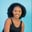Related Tags

pandas
python3
python

# How to use the Series.ewm() method in pandasMaria Elijah

## Overview

The Series.ewm() function in the pandas library in Python offers exponentially weighted (EW) function calculations.

## Syntax

Series.ewm(self, com=None, span=None, halflife=None, alpha=None, min_periods=0, adjust=True, ignore_na=False, axis=0)
• com: This represents the decay in terms of the center of mass, α=1/(1+com), for com≥0.

• span: This represents the decay in terms of the span, α=2/(span+1), for span≥1.

• halflife: This represents the decay in terms of the half-life, α=1−exp(log(0.5)/halflife), for halflife>0.

• alpha: This indicates smoothing factor α, 0<α≤1.

• min_periods: This represents the minimum number of observations needed to have a value in the window. The default value is 0.

• adjust: This is divided by the decaying adjustment factor into the initial periods to consider the imbalance in the relative weightings (looking at the EWMA as a moving average).

• ignore_na: This specifies that the missing values should be ignored when calculating weights. The default is False.

• axis: The default value is 0. It specifies the axis on which the function is to be performed. If the value is 0, the operation is performed across the rows. Otherwise, the operation is performed across the columns.

## Code example

The following code demonstrates how to use the Series.ewm() function in pandas:

import pandas as pd

# Creating the series
my_series=pd.Series(range(1,20,5), index=[x for x in 'shot'])

# Printing the series
print(my_series)

# Using Series.ewm()
print(my_series.ewm(com=0.5, adjust=True).mean())

### Code explanation

• Line 1: We import the pandas library.

• Line 4: Using the range() function, we create a series and set the index to shot.

• Line 7: The series elements are displayed.

• Lines 10: We calculate the exponential weight of elements in the series using the ewm() function and set the com and adjust parameters.

RELATED TAGS

pandas
python3
python

CONTRIBUTORMaria Elijah
RELATED COURSES

View all Courses

Keep Exploring

Learn in-demand tech skills in half the time